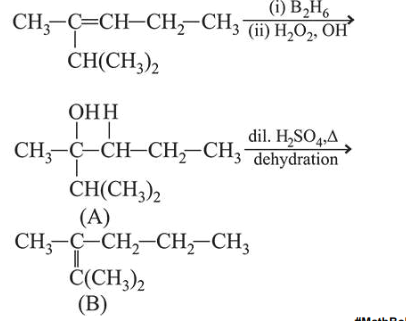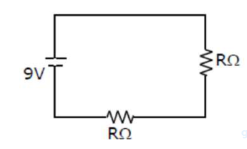# Figure shows a circuit that contains four identical resistors with resistance

Question:

Figure shows a circuit that contains four identical resistors with resistance $\mathrm{R}=2.0 \Omega$. TWo identical inductors with

inductance $\mathrm{L}=2.0 \mathrm{mH}$ and an ideal battery with emf $\mathrm{E}=9$. V. The current 'i' just after the switch 's' is closed will be :1. (1) $9 \mathrm{~A}$

2. (2) $3.0 \mathrm{~A}$

3. (3) $2.25 \mathrm{~A}$

4. (4) $3.37 \mathrm{~A}$

Correct Option: , 3

Solution:

(3)

When switch $S$ is closed-

Given : $v=9 v$

From $V=I R$

$I=\frac{V}{R}$

$R_{\mathrm{eq} .}=2+2=4 \Omega$

$I=\frac{9}{4}=2.25 \mathrm{~A}$